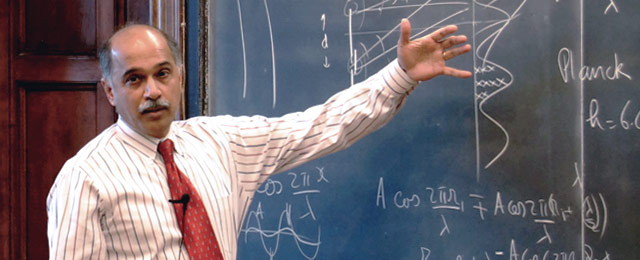# Fundamentals of Physics ICourse Number
PHYS 200

This course provides a thorough introduction to the principles and methods of physics for students who have good preparation in physics and mathematics. Emphasis is placed on problem solving and quantitative reasoning. This course covers Newtonian mechanics, special relativity, gravitation, thermodynamics, and waves.

Course Structure

This Yale College course, taught on campus twice per week for 75 minutes, was recorded for Open Yale Courses in Fall 2006. The Open Yale Courses Book Series. For more information about Professor Shankar's book based on the lectures from this course, Fundamentals of Physics: Mechanics, Relativity, and Thermodynamics http://yalepress.yale.edu/yupbooks/book.asp?isbn=9780300192209 click here.

### Syllabus

Professor
Description

This course provides a thorough introduction to the principles and methods of physics for students who have good preparation in physics and mathematics. Emphasis is placed on problem solving and quantitative reasoning. This course covers Newtonian mechanics, special relativity, gravitation, thermodynamics, and waves.

Texts

Wolfson, Richard and Jay Pasachoff. 1998. Physics with Modern Physics for Scientists and Engineers, 3d ed. Reading, MA: Addison Wesley Publishing Company.

Recommended: Shankar, Ramamurti. 2003. Basic Training in Mathematics: A Fitness Program for Science Students. New York: Springer Publishing Company.

Requirements

Homework will be given every Wednesday and is due the following Wednesday before class. Solutions will be posted on Wednesdays, so problem sets must be turned in on time. There will be one in-class midterm halfway through the semester.

Homework: 20%
Midterm examination: 30%
Final examination: 50%
Letter grades will be assigned by taking the highest score of either the total final average or the final exam.

### Sessions

 Lecture 1 Course Introduction and Newtonian Mechanics Lecture 2 Vectors in Multiple Dimensions Lecture 3 Newton's Laws of Motion Lecture 4 Newton's Laws (cont.) and Inclined Planes Lecture 5 Work-Energy Theorem and Law of Conservation of Energy Lecture 6 Law of Conservation of Energy in Higher Dimensions Lecture 8 Dynamics of a Multiple-Body System and Law of Conservation of Momentum Lecture 9 Rotations, Part I: Dynamics of Rigid Bodies Lecture 10 Rotations, Part II: Parallel Axis Theorem Lecture 11 Torque Exam 1 Midterm Exam Lecture 12 Introduction to Relativity Lecture 13 Lorentz Transformation Lecture 14 Introduction to the Four-Vector Lecture 15 Four-Vector in Relativity Lecture 16 The Taylor Series and Other Mathematical Concepts Lecture 17 Simple Harmonic Motion Lecture 18 Simple Harmonic Motion (cont.) and Introduction to Waves Lecture 20 Fluid Dynamics and Statics and Bernoulli's Equation Lecture 21 Thermodynamics Lecture 22 The Boltzmann Constant and First Law of Thermodynamics Lecture 23 The Second Law of Thermodynamics and Carnot's Engine Lecture 24 The Second Law of Thermodynamics (cont.) and Entropy Exam 2 Final Exam

### Survey